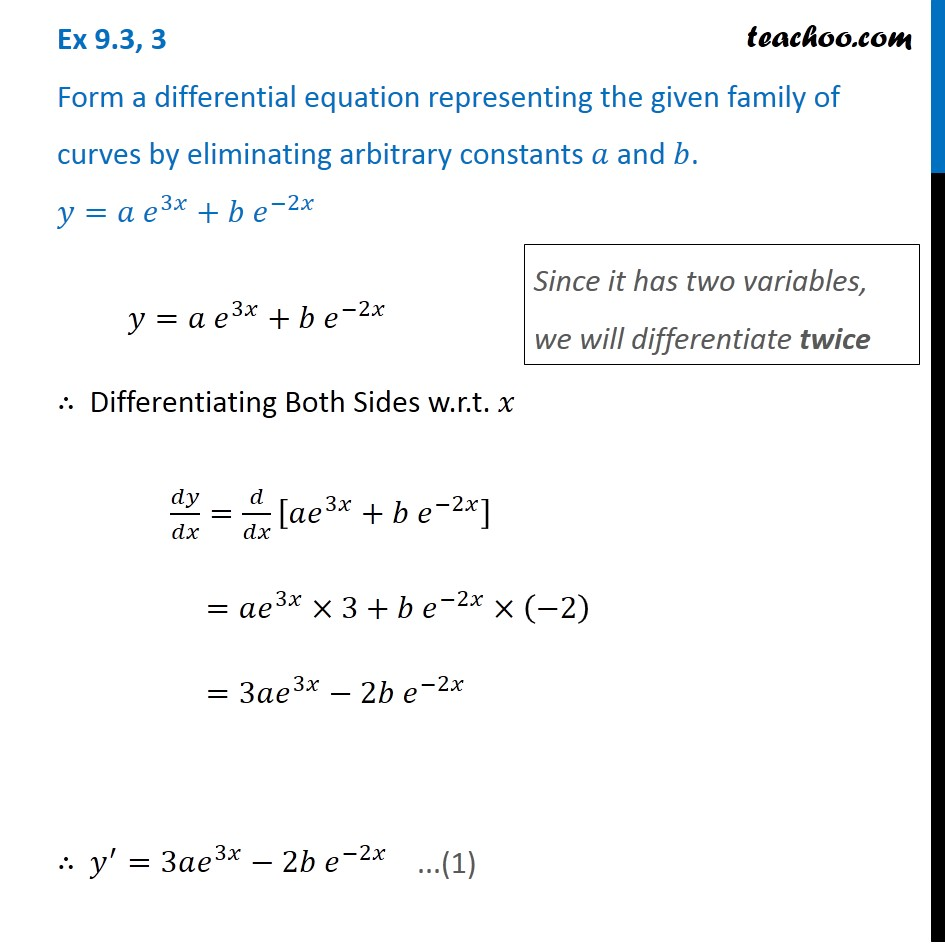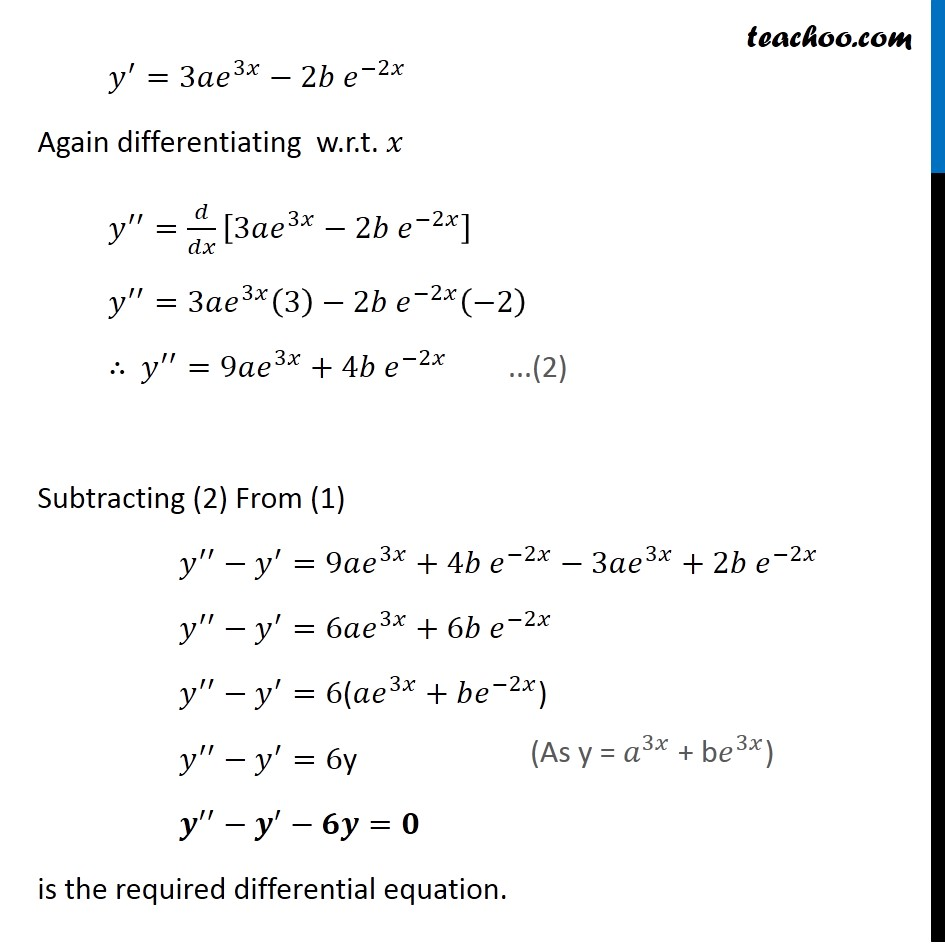Forming Differential equations

Chapter 9 Class 12 Differential Equations
Serial order wiseLearn in your speed, with individual attention - Teachoo Maths 1-on-1 Class

### Transcript

Question 3 Form a differential equation representing the given family of curves by eliminating arbitrary constants 𝑎 and 𝑏. 𝑦=𝑎 𝑒^3𝑥+𝑏 𝑒^(−2𝑥) Since it has two variables, we will differentiate twice 𝑦=𝑎 𝑒^3𝑥+𝑏 𝑒^(−2𝑥) ∴ Differentiating Both Sides w.r.t. 𝑥 𝑑𝑦/𝑑𝑥=𝑑/𝑑𝑥 [𝑎𝑒^3𝑥+𝑏 𝑒^(−2𝑥) ] =𝑎𝑒^3𝑥×3+𝑏 𝑒^(−2𝑥)×(−2) =3𝑎𝑒^3𝑥−2𝑏 𝑒^(−2𝑥) ∴ 𝑦^′=3𝑎𝑒^3𝑥−2𝑏 𝑒^(−2𝑥) ...(1) 𝑦^′=3𝑎𝑒^3𝑥−2𝑏 𝑒^(−2𝑥) Again differentiating w.r.t. 𝑥 𝑦^′′=𝑑/𝑑𝑥 [3𝑎𝑒^3𝑥−2𝑏 𝑒^(−2𝑥) ] 𝑦^′′=3𝑎𝑒^3𝑥 (3)−2𝑏 𝑒^(−2𝑥) (−2) ∴ 𝑦^′′=9𝑎𝑒^3𝑥+4𝑏 𝑒^(−2𝑥) Subtracting (2) From (1) 𝑦^′′−𝑦^′=9𝑎𝑒^3𝑥+4𝑏 𝑒^(−2𝑥)−3𝑎𝑒^3𝑥+2𝑏 𝑒^(−2𝑥) 𝑦^′′−𝑦^′=6𝑎𝑒^3𝑥+6𝑏 𝑒^(−2𝑥) 𝑦^′′−𝑦^′=6(𝑎𝑒^3𝑥+𝑏𝑒^(−2𝑥)) 𝑦^′′−𝑦^′=6y 𝒚^′′−𝒚^′−𝟔𝒚=𝟎 is the required differential equation. (As y = 𝑎^3𝑥 + b𝑒^3𝑥)